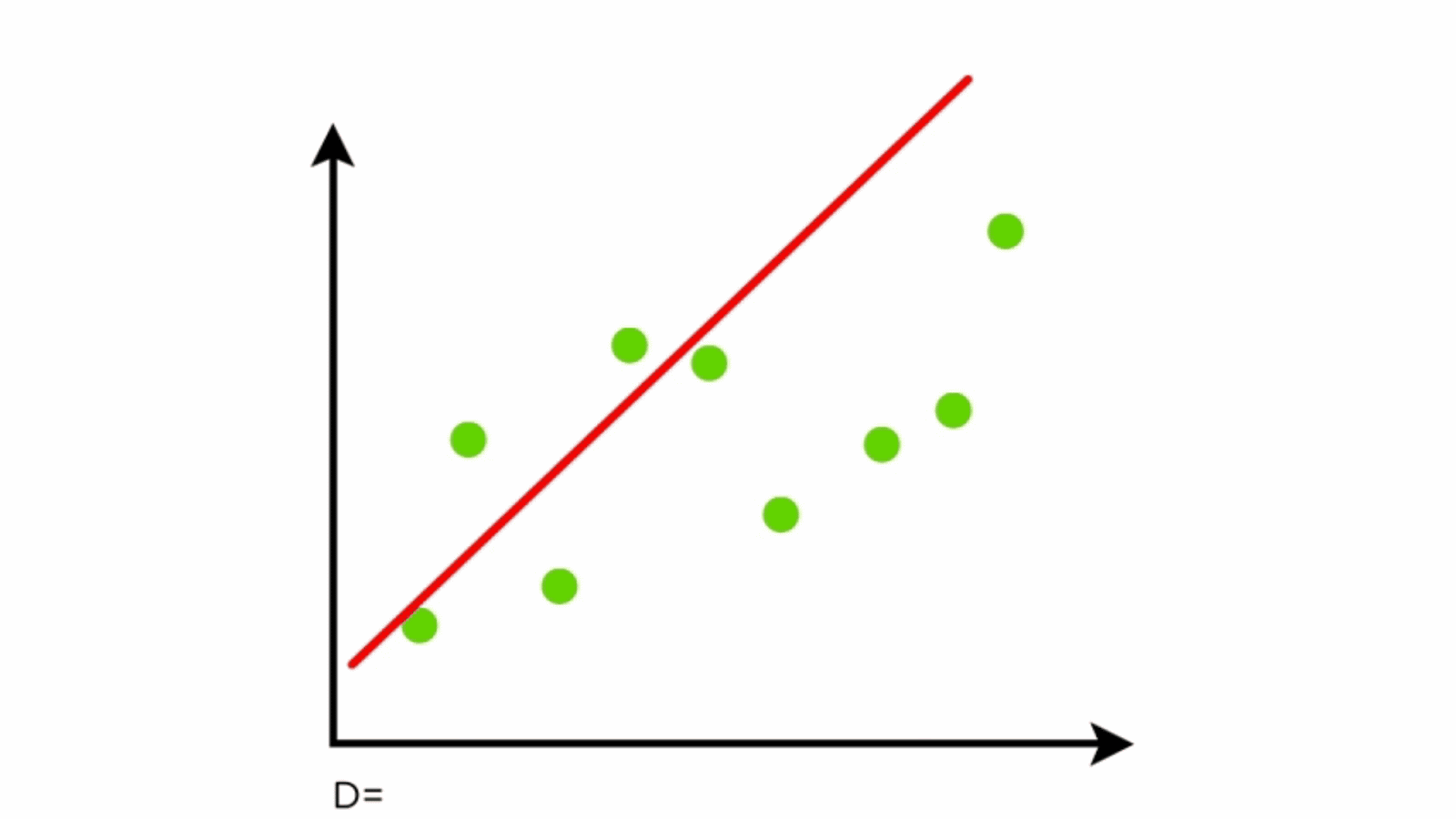# My Understanding of Linear Regression

`from sklearn.linear_model import LinearRegressionmodel = LinearRegression(fit_intercept=True)rng = np.random.RandomState(1)X = 10 * rng.rand(100, 3)y = 0.5 + np.dot(X, [1.5, -2., 1.])+0.1*rng.randn(100)model.fit(X, y)print(model.intercept_)print(model.coef_)`
`0.5156233346576982[ 1.49815954 -1.99762243  0.99725804]`
• response (Y): variable to predict
• independent variable (X): the variable used to predict the response
• record (x, y): one observation
• intercept: the response Y when independent variable X is zero
• least squares: the method of fitting a regression by minimizing the sum of squared residuals, and least squares method is sensitive to outliers
• Root Mean Squared Error (RMSE): the square root of the average squared error of the regression. It has the very similar meaning with Residual Standard Error (RSE).
`from sklearn.metrics import r2_score, mean_squared_errorRMSE = np.sqrt(mean_squared_error(gd, predicted))`
• R2 is a statistic that will give some information about the goodness of fit of a model. In regression, the R2 coefficient of determination is a statistical measure of how well the regression predictions approximate the real data points. An R2 of 1 indicates that the regression predictions perfectly fit the data. It is defined as the proportion of variation in the data that is accounted for in the model.
`from sklearn.metrics import r2_score, mean_squared_errorr2_score(gd, predicted)`
• t-statistics: it is opposite to p-value. The larger it is, the more important it is. High t-statistics indicate the model should contain this variable while low t-statistics shows the model should discard this variable. t-statistics is given when using statismodels to set up a linear regression model.
`predictors = ['SqFtTotLiving', 'SqFtLot', 'Bathrooms',               'Bedrooms', 'BldgGrade']outcome = 'AdjSalePrice'house_lm = LinearRegression()house_lm.fit(house[predictors], house[outcome])print(f'Intercept: {house_lm.intercept_:.3f}')print('Coefficients:')for name, coef in zip(predictors, house_lm.coef_):    print(f' {name}: {coef}')`
`import statismodels.api as smmodel = sm.OLS(house[outcome], house[predictors].assign(const=1))results = model.fit()print(results.summary())`
• Practical Statistics for Data Scientists 50+ Essential Concepts Using R and Python: CHAPTER 4 Regression and Prediction

--

--

--

## More from ifeelfree

Love podcasts or audiobooks? Learn on the go with our new app.

## Get your team started on Computer Vision## Process followed in Data Science projects at high level## Stop Asking People If They’ve Lost Weight## Paper Summary: BLIP: Bootstrapping Language-Image Pre-training for Unified Vision-Language…## Money laundering remains a major risk for banks (and not only for the Nordics)## ICLR 2018 Posters Highlight (part 1)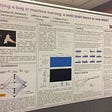## Linear Regression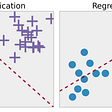## Acoustic Features for Neural Vocoders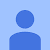## Introduction to Data Science and Understanding Linear Regression in a simpler way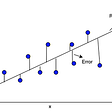## Linear Regression : decoded## Linear Regression## LINEAR REGRESSION